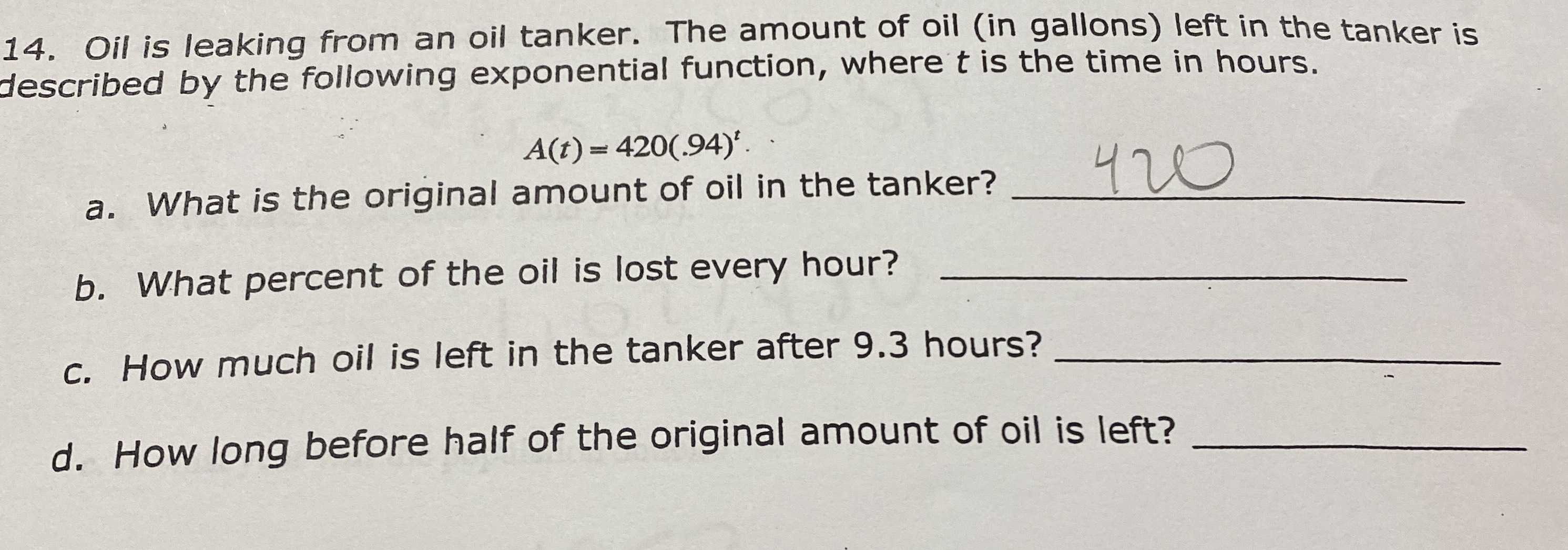### ¿Todavía tienes preguntas de matemáticas?

Pregunte a nuestros tutores expertos
Algebra
Pregunta14. Oil is leaking from an oil tanker. The amount of oil (in gallons) left in the tanker is described by the following exponential function, where $$t$$ is the time in hours.

$$A ( t ) = 420 ( .94 ) ^ { t } .$$